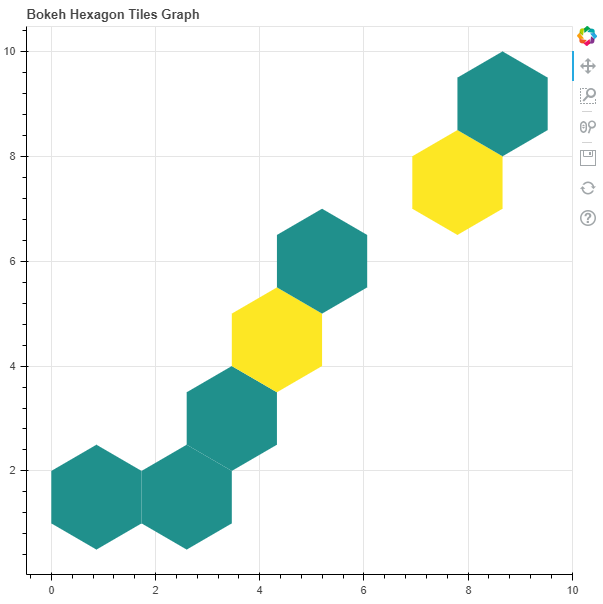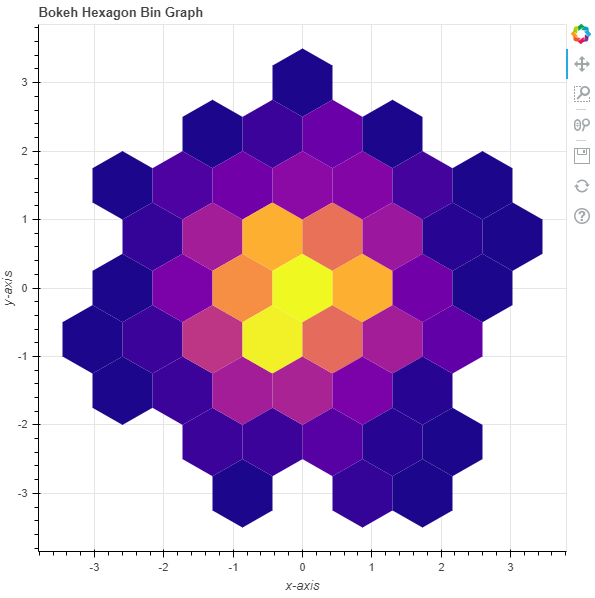Open in App
Not now

# Python Bokeh – Plotting Hexagon Bins on a Graph

• Last Updated : 10 Jul, 2020

Bokeh is a Python interactive data visualization. It renders its plots using HTML and JavaScript. It targets modern web browsers for presentation providing elegant, concise construction of novel graphics with high-performance interactivity.

Bokeh can be used to plot hexagon tiles on a graph. Plotting hexagon tiles on a graph can be done using the `hexbin()` method of the `plotting` module.

## plotting.figure.hexbin()

Syntax : hexbin(parameters)

Parameters :

• x : NumPy array of x-coordinates of the hexagon tile bins
• y : NumPy array of y-coordinates of the hexagon tile bins
• size : radius of the hexagonal tile bins, default is 1
• palette : orientation value, default is Viridis256
• orientation : palette to colormap the bins, default is pointytop
• line_color : color of the line, default is black
• fill_color : fill color value of the hexagon tile bin markers
• aspect_scale : aspect ratio scaling value

Any additional keyword arguments are passed to `hex_tile()`.

Returns : an object of class `GlyphRenderer` and `DataFrame`

Example 1 : In this example we will be using the default values for plotting the graph.

 `# importing the modules ``from` `bokeh.plotting ``import` `figure, output_file, show ``import` `numpy as np`` ` `# file to save the model ``output_file(``"gfg.html"``) ``      ` `# instantiating the figure object ``graph ``=` `figure(title ``=` `"Bokeh Hexagon Bin Graph"``) ``   ` `# the points to be plotted ``x ``=` `np.array([``1``, ``2``, ``3``, ``4``, ``5``, ``6``, ``7``, ``8``, ``9``])``y ``=` `np.array([``1``, ``2``, ``3``, ``4``, ``5``, ``6``, ``7``, ``8``, ``9``])`` ` `# plotting the graph ``graph.hexbin(x, y, size ``=` `1``) ``    ` `# displaying the model ``show(graph) `

Output :Example 2 : In this example we will be plotting the hexagon bins with various parameters

 `# importing the modules ``from` `bokeh.plotting ``import` `figure, output_file, show ``import` `numpy as np`` ` `# file to save the model ``output_file(``"gfg.html"``) ``      ` `# instantiating the figure object ``graph ``=` `figure(title ``=` `"Bokeh Hexagon Bin Graph"``) `` ` `# name of the x-axis ``graph.xaxis.axis_label ``=` `"x-axis"``      ` `# name of the y-axis ``graph.yaxis.axis_label ``=` `"y-axis"``   ` `# the points to be plotted ``x ``=` `np.random.standard_normal(``500``)``y ``=` `np.random.standard_normal(``500``)`` ` `# size of the bins``size ``=` `0.5`` ` `# color palette of the graph``palette ``=` `"Plasma256"`` ` `# plotting the graph ``graph.hexbin(x, y,``             ``size ``=` `0.5``,``             ``palette ``=` `palette) ``    ` `# displaying the model ``show(graph)  `

Output :My Personal Notes arrow_drop_up